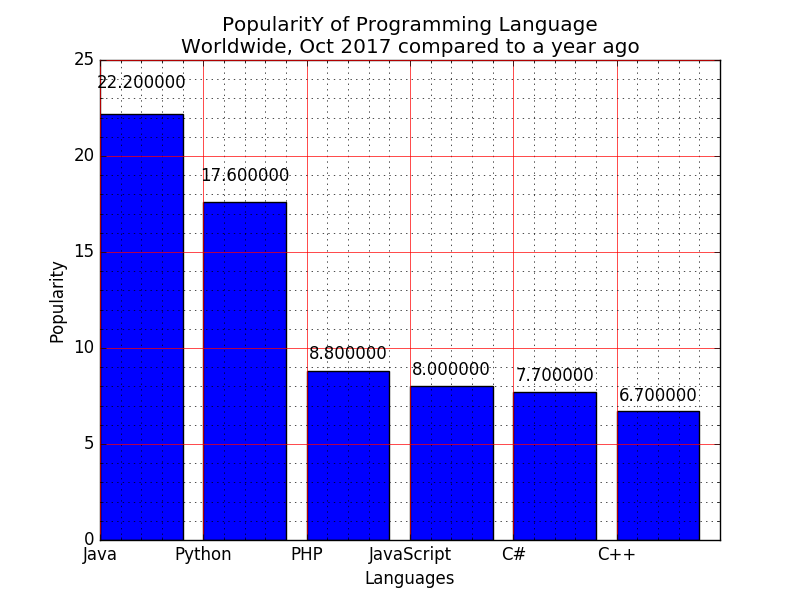﻿ Matplotlib Bar Chart: Display a bar chart of the popularity of programming Languages and attach a text label above each bar displaying its popularity - w3resource# Matplotlib Bar Chart: Display a bar chart of the popularity of programming Languages and attach a text label above each bar displaying its popularity

## Matplotlib Bar Chart: Exercise-5 with Solution

Write a Python programming to display a bar chart of the popularity of programming Languages. Attach a text label above each bar displaying its popularity (float value).

Sample data:
Programming languages: Java, Python, PHP, JavaScript, C#, C++
Popularity: 22.2, 17.6, 8.8, 8, 7.7, 6.7

Sample Solution:

Python Code:

``````import matplotlib.pyplot as plt
x = ['Java', 'Python', 'PHP', 'JavaScript', 'C#', 'C++']
popularity = [22.2, 17.6, 8.8, 8, 7.7, 6.7]
x_pos = [i for i, _ in enumerate(x)]

fig, ax = plt.subplots()
rects1 = ax.bar(x_pos, popularity, color='b')
plt.xlabel("Languages")
plt.ylabel("Popularity")
plt.title("PopularitY of Programming Language\n" + "Worldwide, Oct 2017 compared to a year ago")
plt.xticks(x_pos, x)
# Turn on the grid
plt.minorticks_on()
plt.grid(which='major', linestyle='-', linewidth='0.5', color='red')
# Customize the minor grid
plt.grid(which='minor', linestyle=':', linewidth='0.5', color='black')
def autolabel(rects):
"""
Attach a text label above each bar displaying its height
"""
for rect in rects:
height = rect.get_height()
ax.text(rect.get_x() + rect.get_width()/2., 1.05*height,
'%f' % float(height),
ha='center', va='bottom')
autolabel(rects1)

plt.show()
```
```

Sample Output:Python Code Editor: